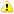# MONTHS_BETWEEN Function - Oracle to MariaDB Migration

In Oracle, MONTHS_BETWEEN(date1, date2) function returns the number of months between two dates as a decimal number.

Note that MariaDB TIMESTAMPDIFF(month, date2, date1) function does not return exactly the same result, and you have to use an user-defined function if you need to fully emulate the Oracle MONTHS_BETWEEN function (see UDF's code below).

Oracle:

```  ALTER SESSION SET NLS_DATE_FORMAT = 'YYYY-MM-DD';

-- 1-day difference
SELECT MONTHS_BETWEEN('2021-03-01', '2021-02-28') FROM dual;
# 0.129032258

-- Still 1-day difference but the result is different
SELECT MONTHS_BETWEEN('2021-03-02', '2021-03-01') FROM dual;
# 0.32258065```

TIMESTAMPDIFF always returns an integer result:

```  -- 1-day difference
SELECT TIMESTAMPDIFF(month, '2021-02-28', '2021-03-01');
# 0

-- 1-day difference
SELECT TIMESTAMPDIFF(month, '2021-03-01', '2021-03-02');
# 0```

Also note that MONTHS_BETWEEN and TIMESTAMPDIFF have different order of parameters.## Oracle MONTHS_BETWEEN in Detail

MONTHS_BETWEEN returns the number of full months between dates and a fractional part.

An integer value is returned only if:

• Both dates specify the same day of the month (February 13 and March 13 i.e.)
• Both dates are the last days of the months (January 31 and April 30 i.e.)

Oracle:

```  -- Between March 13 and February 13
SELECT MONTHS_BETWEEN('2021-03-13', '2021-02-13') FROM dual;
# 1

-- Between April 30 and January 31
SELECT MONTHS_BETWEEN('2021-04-30', '2021-01-31') FROM dual;
# 3```

Fractional Part

The fractional part is calculated using the following formula:

 Condition Fractional Part Calculation If day_of_date1 > day_of_date2 (day_of_date1 - day_of_date2) / 31 If day_of_date1 < day_of_date2 (31 - day_of_date2 + day_of_date1) / 31

Note that when MONTHS_BETWEEN calculates the fractional part, it considers that all months have 31 days.

Consider the following examples:

Oracle:

``` -- 1-day difference
SELECT MONTHS_BETWEEN('2021-03-01', '2021-02-28') FROM dual;
# 0.129032258```

Although there is just 1-day difference between February 28, 2021 and March 01, 2021, MONTHS_BETWEEN considers Feb 29, Feb 30, Feb 31 and Mar 01:

 (31 - 28 + 1) / 31 = 0.129032258

Another example:

```  -- Still 1-day difference but the result is different
SELECT MONTHS_BETWEEN('2021-03-02', '2021-03-01') FROM dual;
# 0.32258065```

Now the fractional part is calculated as follows:

 (2 - 1) / 31 = 0.32258065

## MariaDB User-Defined Function to Emulate Oracle MONTHS_BETWEEN

You can use the following user-defined function to emulate Oracle MONTHS_BETWEEN function:

```  DELIMITER //

CREATE FUNCTION MONTHS_BETWEEN (p_date1 DATETIME, p_date2 DATETIME)
RETURNS FLOAT
DETERMINISTIC
BEGIN
DECLARE months FLOAT DEFAULT TIMESTAMPDIFF(month, p_date2, p_date1);

-- Both dates does not point to the same day of month
IF DAY(p_date1) <> DAY(p_date2) AND
-- Both dates does not point to the last day of month
(MONTH(p_date1) = MONTH(p_date1 + INTERVAL 1 DAY) OR
MONTH(p_date2) = MONTH(p_date2 + INTERVAL 1 DAY))
THEN
-- Correct to include full months only and calculate fraction
IF DAY(p_date1) < DAY(p_date2) THEN
SET months = months + CONVERT(31 - DAY(p_date2) + DAY(p_date1), FLOAT) / 31;
ELSE
SET months = months + CONVERT(DAY(p_date1) - DAY(p_date2), FLOAT) / 31;
END IF;
END IF;

RETURN months;
END;
//

DELIMITER ;```

Now you can use the UDF as follows:

```    -- 1-day difference